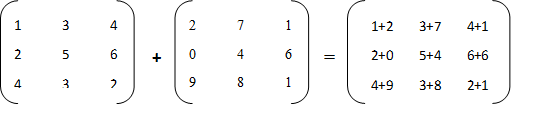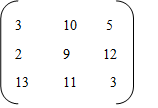# Java program to add two matrices

By | December 22, 2018

In this post of Java program to add two matrices we are going to learn how we can write a java program to add two matrices.

When we add two matrices addition is done index based we add the element at (0, 0) in the first matrix with the element at (0, 0) in the second matrix, element at (0, 1) in the first matrix with the element at (0, 1) in the second matrix and so on.

For example – If you are adding two matrices of order 3X3Thus the resultant matrix is –We should also remember these points when adding one matrix with another –

1. Both of the matrices have to be of same size or dimension.
2. Resultant matrix will also have the same order for the elements. Element at (0, 0) in the first matrix added with (0, 0) of the second matrix becomes the element at index (0, 0) in the resultant matrix too.

java program to add two matrices

Output of above program for 3X3 matrices:

That’s all for this topic Java program to adds two matrices. If you have any questions or doubts or any suggestions to make please drop a comment.